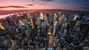Announcements
Applications are open for the 2024 Qlik Luminary Program. Apply by December 15 here.
cancel
Showing results for
Did you mean:Contributor II

## Using column() in another calculated column (sum) within a straight table

I currently have a problem using column() for another, calculated column within a straight table. I tried to recreate a simplified version of the problem to explain it:

Assuming I have the following data loaded in my app:

 date1 val1 01/05/2023 A 01/05/2023 A 01/05/2023 A 01/06/2023 A 01/06/2023 A 01/06/2023 B 01/07/2023 B 01/07/2023 C 01/07/2023 C

and I have a straight table in my worksheet with the following columns:

Column 1: Dimension val1

Column 2: Measure with the minimum date for each value in the val1 column plus 1 month, i.e. addMonths(date(min(date1)), 1)  (Note: in my actual app, I add a variable number of months which can be set by the user via a variable input, rather than a fixed value of 1 month)

I would like to create another measure column (Column 3), which displays how often val1 occurs for the date calculated in Column 2.

This means that I would expect the straight table to look like this:

 val1 addMonths(date(min(date1)), 1) Column 3 A 01/06/2023 2 B 01/07/2023 1 C 01/08/2023 0

E.g. "A" occurred twice in the month calculated in column 2.

As Column 2 is dynamic, depending on the value of val1, I believe I can't use a set analysis such as

Count(<{date1 = {'\$(=column(1)'}>} val1)       or

Count(<{date1 = {'\$(=addMonths(date(min(date1)), 1))'}>} val1)   ,

as the set expression is not calculated row-wise but only for all data at once. (column(1) refers to Column 2, as Column 1 in my straight table is a dimension)

As an expression for Column 3, I therefore tried using :

Sum(If(date1 = column(1), 1))           and also

Count(If(date1 = column(1), val1))     , but in both cases column 3 only contains zeroes instead of the values I expected.

I also made sure that this is not a date formatting issue - using date(date1), date(column(1)) or both in the expressions above made no difference in the result. Also using <=, <, >, >= made no difference. Only when using <> it shows something different than zero (number of all occurences per value in val1).

Is there any expression that displays how often val1 occurs for the date calculated in Column 2?

Thanks for your help, which is much appreciated!

Labels (1)
• ### General Question

1 Solution

Accepted SolutionsMVP

I feel like the aggr() approach is correct and I'm just getting something wrong in how I'm structuring it, but in this case, after taking a step back I think there's an easier solution that doesn't actually require aggr().

6 RepliesHi there,

I need to point out a couple of issues in your logic:

1. The function Column() refers to Measures only, not counting the dimensions - so, in your case, you should be referring to Column(1)

2. The function column() cannot be used in Set Analysis. In fact, Set Analysis conditions are evaluated outside of the chart, therefore they cannot be sensitive to any values of chart dimensions or measures. The only condition available in this case is the IF() condition

3. The date in Column 1 is calculated as the minimal date for Val1, plus one month. It is likely that there is no data for Val1, associated with the min(Date) + 1 Month. Maybe you wanted to specify a range of dates - from min date to the min date + 1 month? Otherwise, you will likely to always see zeroes in the new column.

Try to use these suggestions to fix the problem.

Join us at the Masters Summit for Qlik in Orlando or in Dublin to learn advanced Set Analysis, scripting, data modeling, performance, visualizations, and more - delivered by some of the best Qlik experts in the world!MVP

Adding to Oleg's post, which covers most of what you discussed, I'm not sure why you need to reference the column in the first place. You can just plug that column's formula into your other one, using aggr() to tell Qlik what context to evaluate it under.

Sum(If(date1 = aggr(addMonths(date(min(date1)), 1),val1) , 1))Contributor II
Author

I used Column(1) in my expressions in my post above. I can also say for sure that all data  for Val1 has a Date1 value of the first of the month (as in my minimal example), so that there should be corresponding data for the min-date.

@Or  thanks for the suggestion! I tried it but it unfortunately it still shows zero:In case it helps for reproducing the error, here's the load script for my minimal example: (my app uses DD.MM.YYYY as the date format)

let test_date_1 = date('01.05.2023');
let test_date_2 = date('01.06.2023');
let test_date_3 = date('01.07.2023');

[date1, val1
'\$(test_date_1)', A
'\$(test_date_1)', A
'\$(test_date_1)', A
'\$(test_date_2)', A
'\$(test_date_2)', B
'\$(test_date_2)', B
'\$(test_date_3)', B
'\$(test_date_3)', B
'\$(test_date_3)', C
];

And then I just added a straight table with val1 as the first column, addMonths(date(min(date1)),1) as the second column, and now your suggestion Sum(If(date1 = aggr(addMonths(date(min(date1)), 1),val1), 1))  as the third column. And it just shows zero in the third column for me.

I'll try a bit further today, maybe I'll find a way to make it work with Aggr(). I appreciate your help and would be happy to know if you have any further ideas!Contributor II
Author

Also here's a qvf export of my test app if that helpsMVP

I feel like the aggr() approach is correct and I'm just getting something wrong in how I'm structuring it, but in this case, after taking a step back I think there's an easier solution that doesn't actually require aggr().